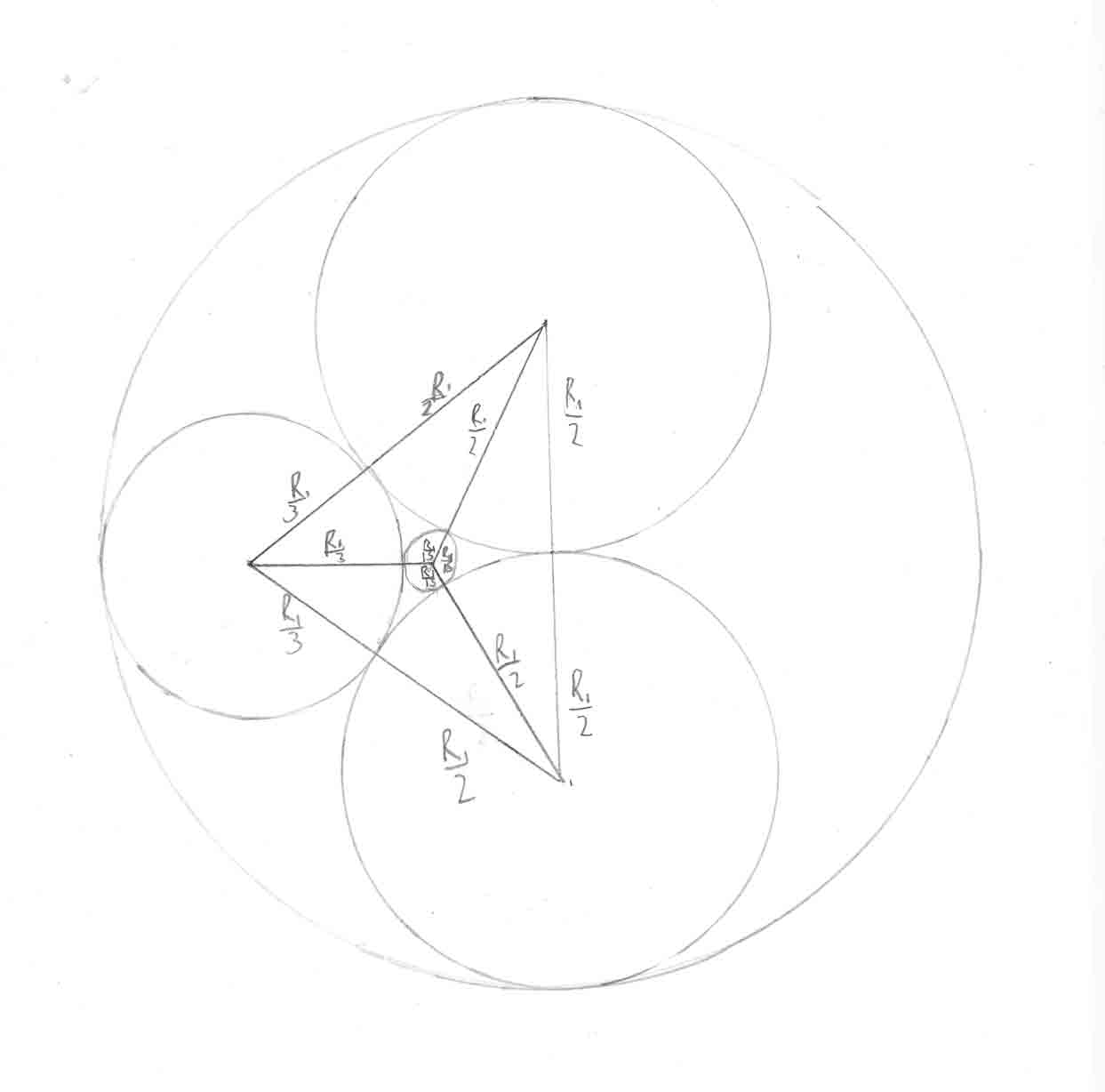Home > Puzzles of the month

# February 2015 - Circles Inside Circles (Solution)

The answer is 225. Let the largest circle have radius R1. The next largest circles have radius R1/2. The second smallest circle's radius must be found using Pythagoras' theorem. Make a triangle with the centres of these circles: The largest circle, one of the next largest circles, and the second smallest circle. Let the second smallest circle have radius R2. The horizontal side length is R1- R2. The vertical side length is R1/2. The hypotenuse has length R1/2+R2. Thus (R1- R2)2+R12/4=(R1/2+R2)2. Expanding, R12- 2R1R2+ R22+ R12/4=R12/4+ R1R2+ R22. Subtracting R12/4 - 2R1R2+ R22, we have R12=3R1R2. Thus R1=3R2 and R2=R1/3. Consider another right-angled triangle with the centres of these circles: The largest circle, one of the second largest circles, and the smallest circle. Let the smallest circle have radius R3. The vertical side is the same. The horizontal side has length R1-2R2- R3. The hypotenuse has length R1/2+R3. Solving in a similar manner to the previous step, R3=R1/15. Now, the smallest circle has area πR12/225=1. Thus πR12=225. However, the largest circle has area πR12. Thus the largest circle has area 225.•The puzzle

•Hall of Fame